# Histograms

 1 What is a Histogram? 2 Histogram Example 3 Histogram Calculator 4 Frequency Histogram 5 Histogram Shapes 6 Difference between Bar Charts & Histograms 7 How to calculate a Histogram? 8 Tips and Tricks on Histogram 9 Solved Examples on Histogram 10 Important Notes on Histogram 11 Practice Questions 12 Maths Olympiad Sample Papers 13 Frequently Asked Questions (FAQs)

We at Cuemath believe that Math is a life skill. Our Math Experts focus on the “Why” behind the “What.” Students can explore from a huge range of interactive worksheets, visuals, simulations, practice tests, and more to understand a concept in depth.

Book a FREE trial class today! and experience Cuemath’s LIVE Online Class with your child.

## What is a Histogram?

A histogram is the graphical representation of data where data is grouped into continuous number ranges and each range corresponds to a vertical bar.

The horizontal axis displays the number range and the vertical axis (frequency) represents the amount of data that is present in each range.

The number ranges depend upon the data that is being used.

## Histogram Example

Uncle Bruno owns a garden with $$30$$ black cherry trees. Each tree is of a different height.The height of the trees (in inches) is given below:

61, 63, 64, 66, 68, 69, 71, 71.5, 72, 72.5, 73, 73.5, 74, 74.5, 76, 76.2, 76.5, 77, 77.5, 78, 78.5, 79, 79.2, 80, 81, 82, 83, 84, 85, 87

We can group the data as follows in a frequency distribution table by setting a range:

Height Range (ft) Number of Trees (Frequency)
60 - 75 3
66 - 70 3
71 - 75 8
76 - 80 10
81 - 85 5
86 - 90 1

This data can be now shown using a histogram.

In a histogram, there shouldn’t be any gaps between the bars.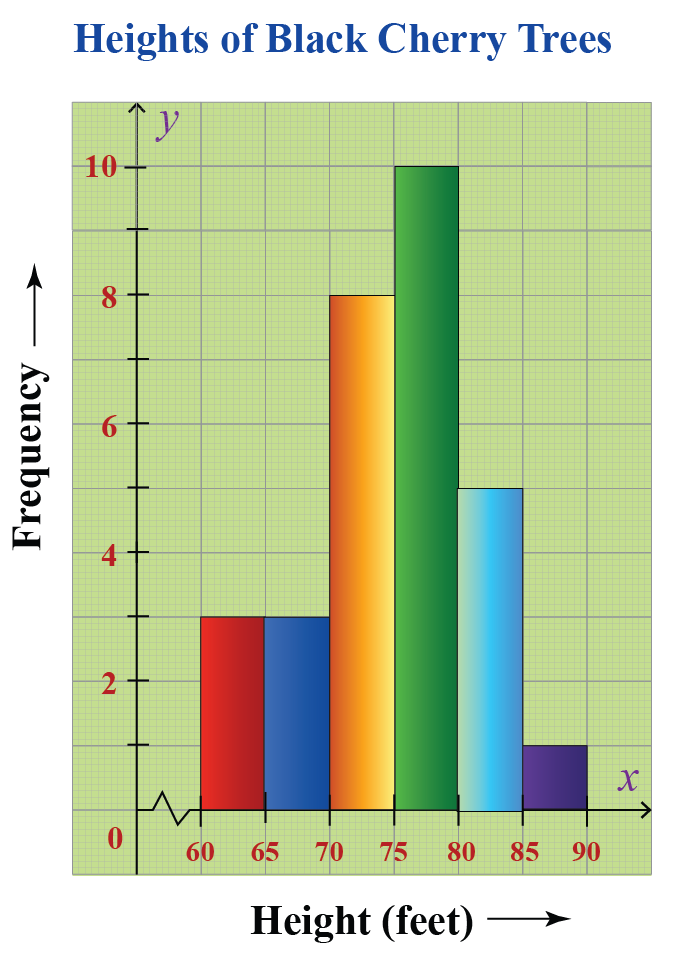CLUEless in Math? Check out how CUEMATH Teachers will explain Histograms to your kid using interactive simulations & worksheets so they never have to memorise anything in Math again!

Explore Cuemath Live, Interactive & Personalised Online Classes to make your kid a Math Expert. Book a FREE trial class today!

## Histogram Calculator

Here is the Histogram Calculator.

Here, we can enter a list of values of data and it gives us the corresponding histogram.

This allows you to change the class interval also by sliding the slider.

## Frequency Histogram

A frequency histogram is a histogram that shows the frequencies (the number of occurrences) of the given data items.

For example;

In a hospital, there are $$20$$ newborn babies whose ages in increasing order are as follows:

1, 1, 1, 1, 2, 2, 2, 2, 2, 3, 3, 3, 3, 3, 3, 3, 3, 4, 4, 5

This information can be shown in a frequency distribution table as follows:

Age (in days) Frequency
$$1$$ $$4$$
$$2$$ $$5$$
$$3$$ $$8$$
$$4$$ $$2$$
$$5$$ $$1$$

This data can be now shown using a frequency histogram.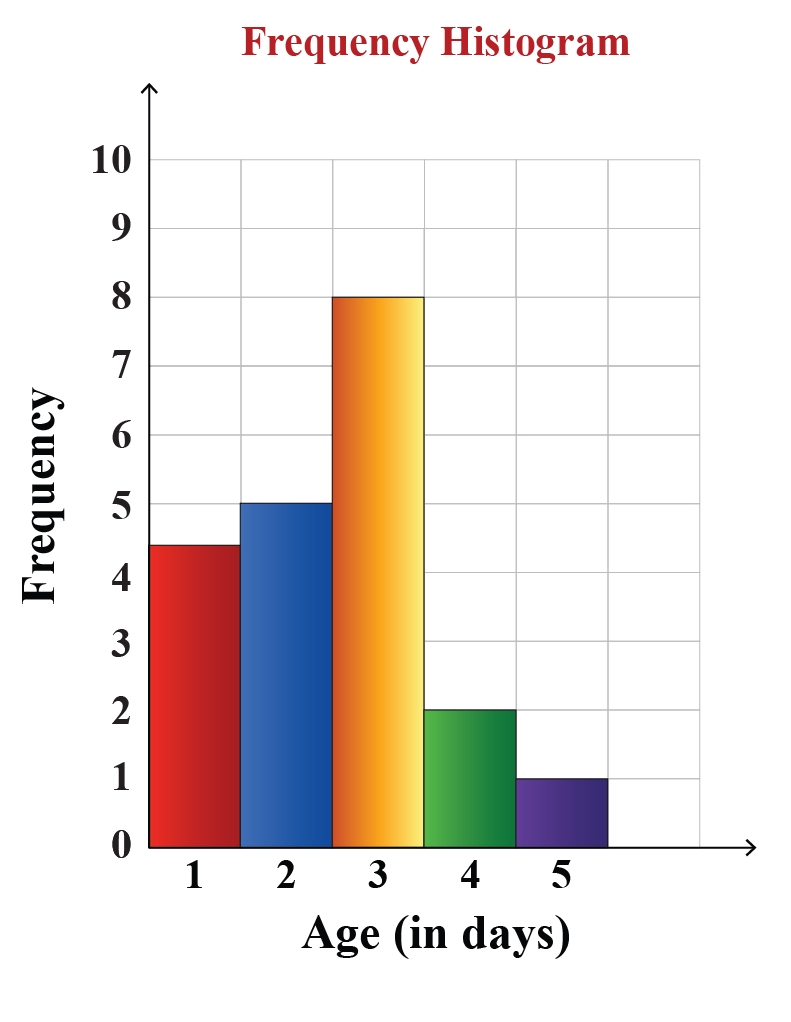## Histogram Shapes

We have mainly $$6$$ types of histogram shapes. They are:

1. Bell Shaped Histogram
2. Bimodal Histogram
3. Skewed Right Histogram
4. Skewed Left Histogram
5. Uniform Histogram
6. Random Histogram

### Bell-Shaped Histogram

A bell-shaped histogram has a single peak.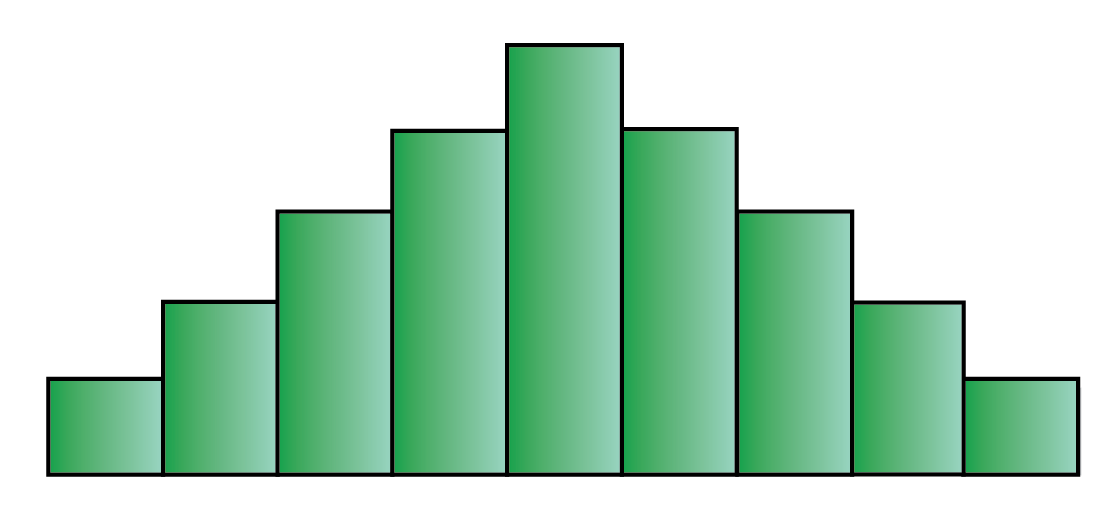Example:

The following histogram shows the number of children visiting a park at different time intervals.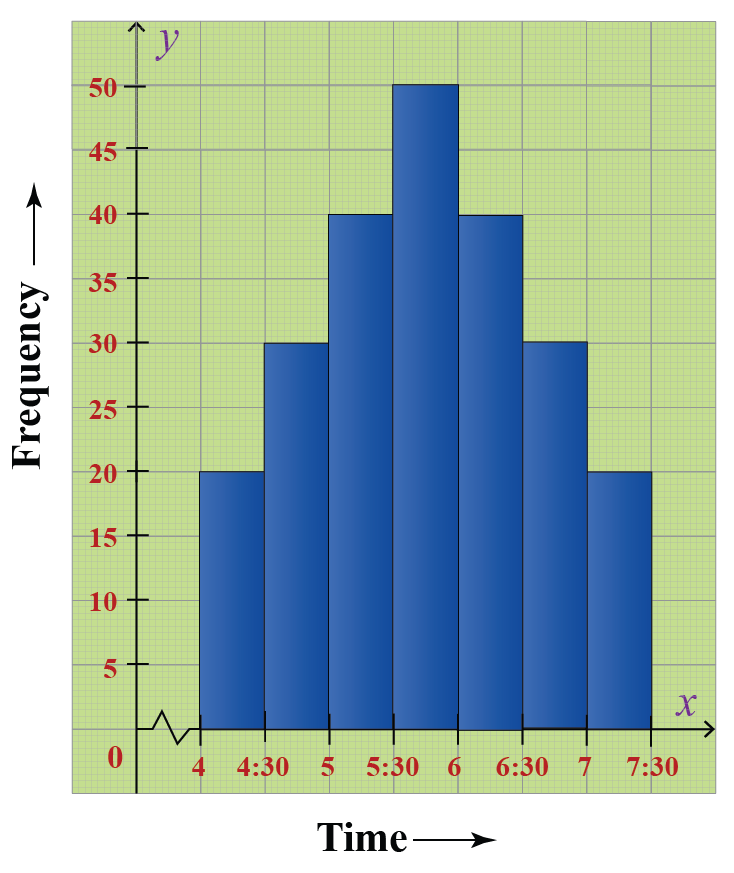The maximum number of children visit the park between 5.30 PM to 6 PM.

The histogram has just one peak at this time interval and hence it is a bell-shaped histogram.

### Bimodal Histogram

A bimodal histogram has two peaks and it looks as follows: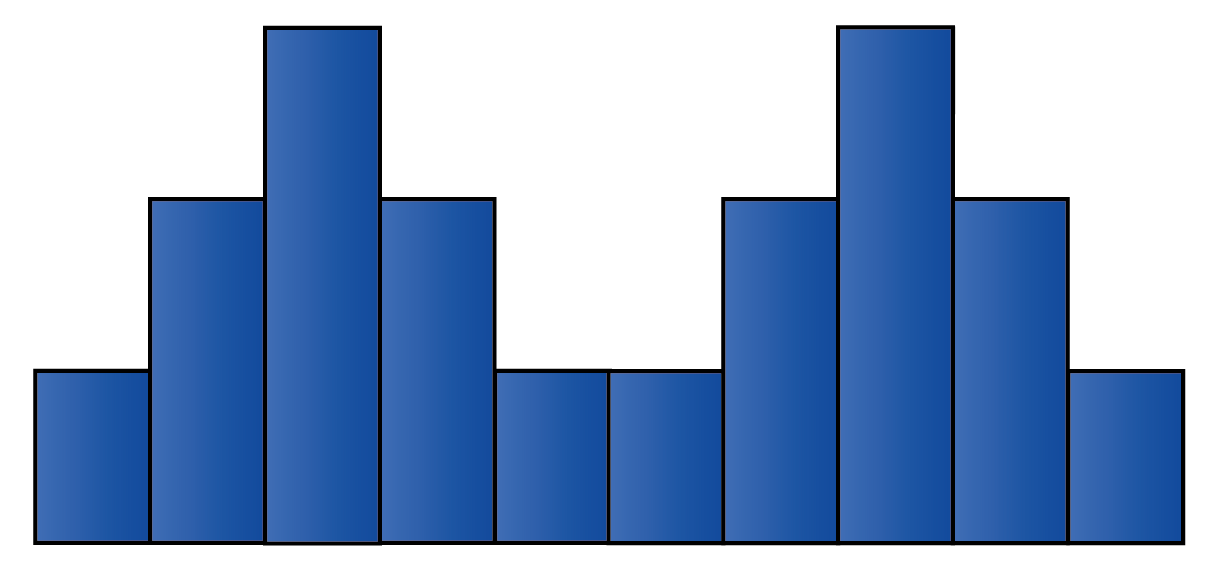Example:

The following histogram shows the marks obtained by the $$48$$ students of Class $$8$$ of St.Mary’s School.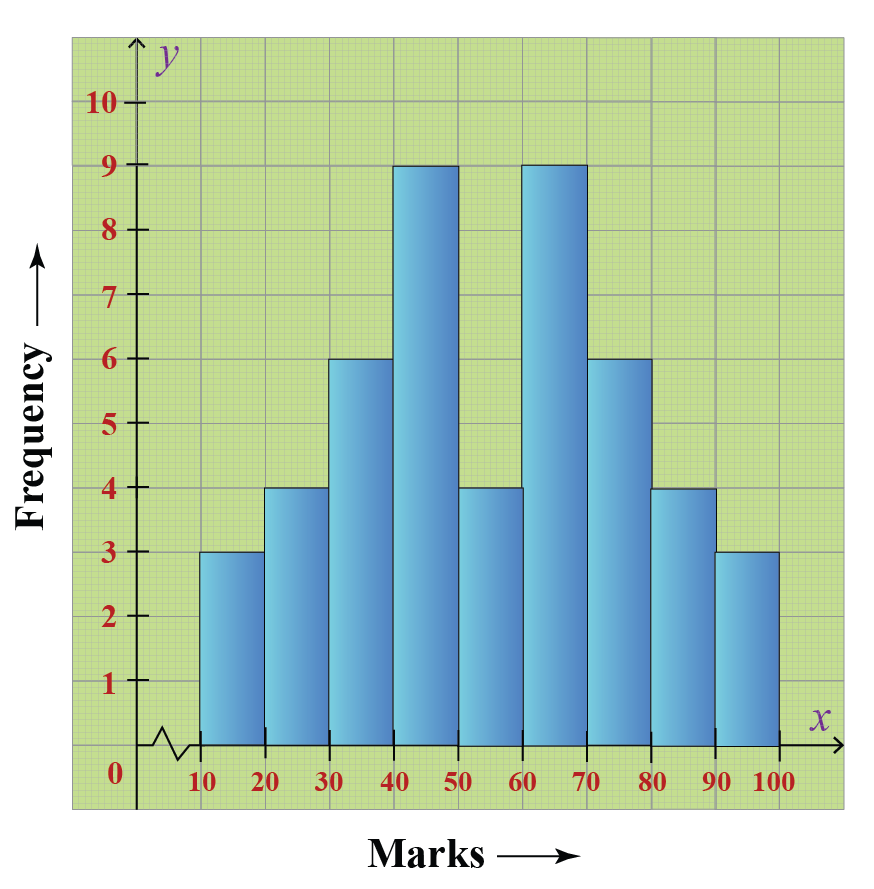The maximum number of students have scored either between 40 to 50 marks OR between 60 to 70 marks.

This histogram has two peaks (between 40 to 50 and between 60 to 70) and hence it is a bimodal histogram.

### Skewed Right Histogram

A skewed right histogram is a histogram that is skewed to the right. It looks as follows: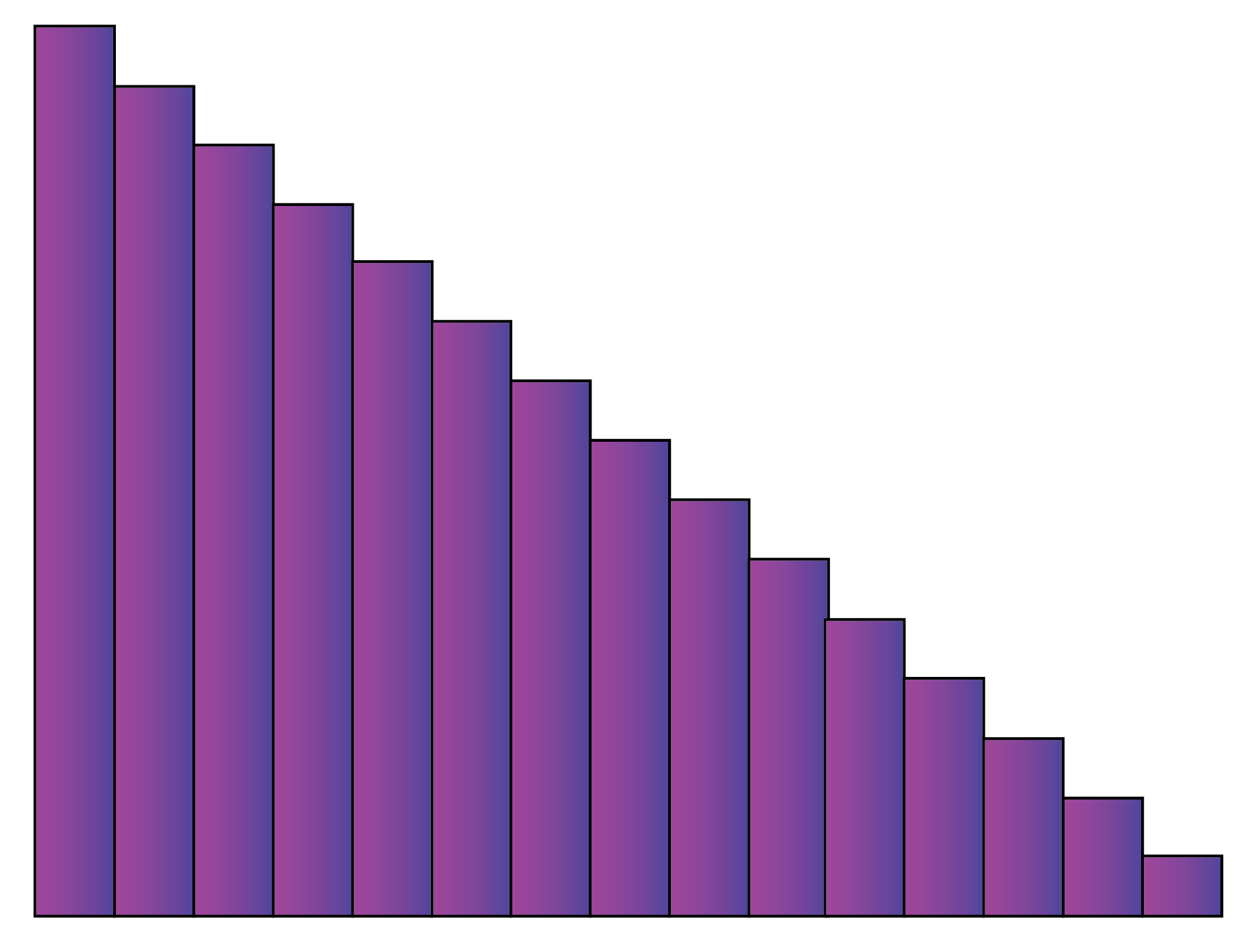Example:

The following histogram shows the number of people corresponding to different wage ranges.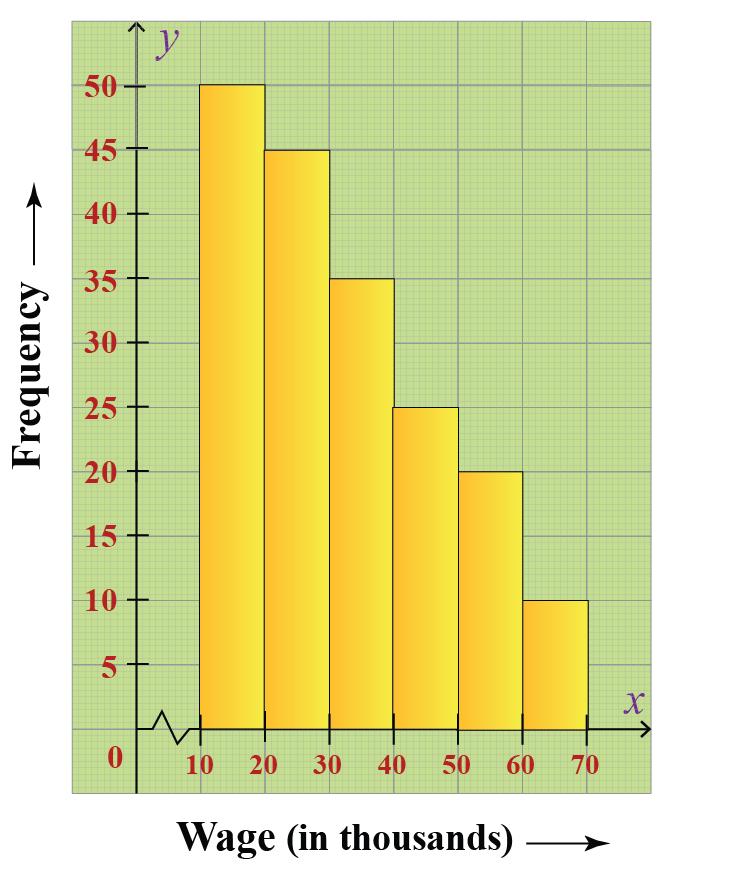Here the bars of the histogram is skewed to the right. Hence, it is a skewed right histogram.

### Skewed Left Histogram

A skewed left histogram is a histogram that is skewed to the left. It looks as follows: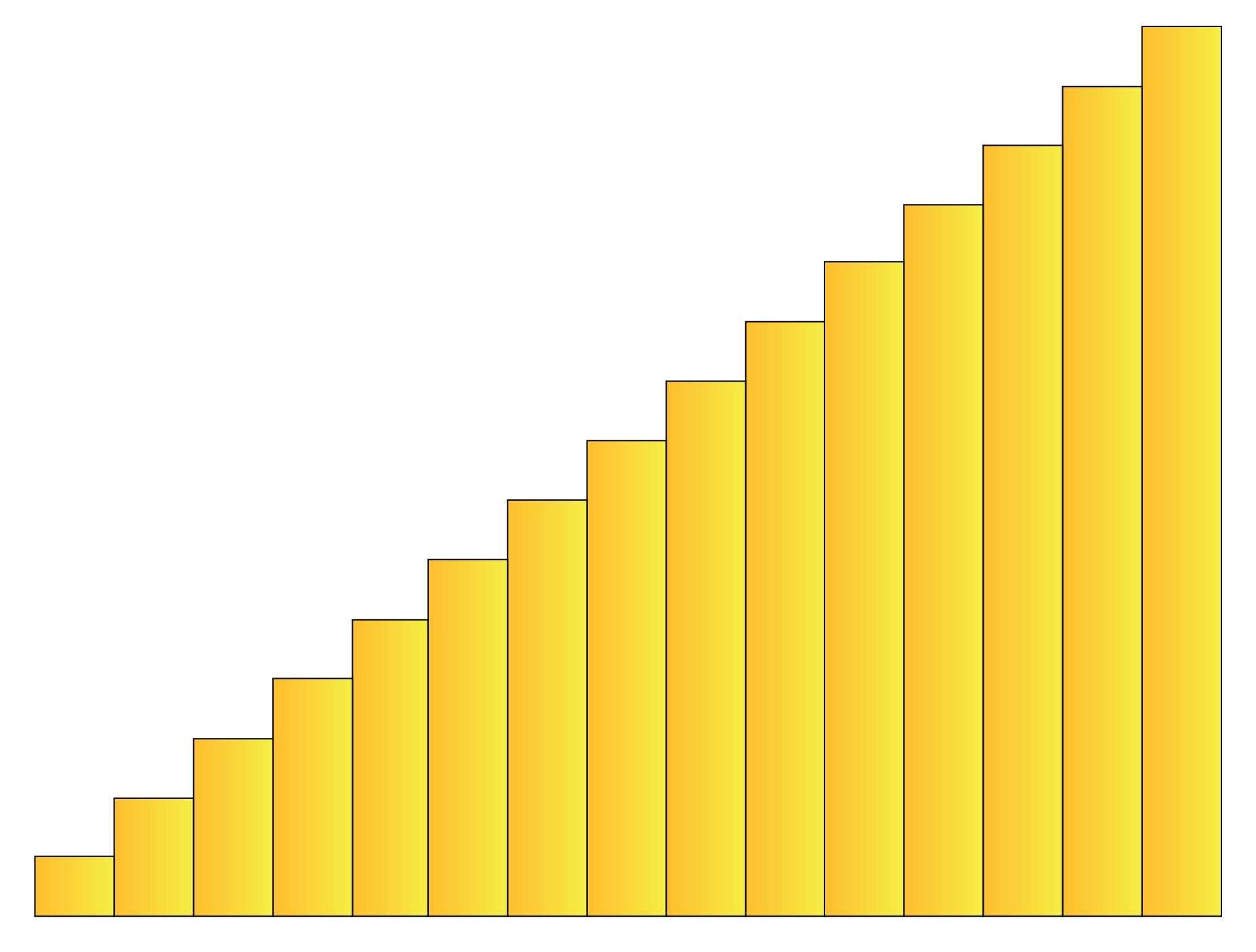Example:

The following histogram shows the number of students of Class $$10$$ of Greenwood High School according to the amount of time they spent on their studies on a daily basis.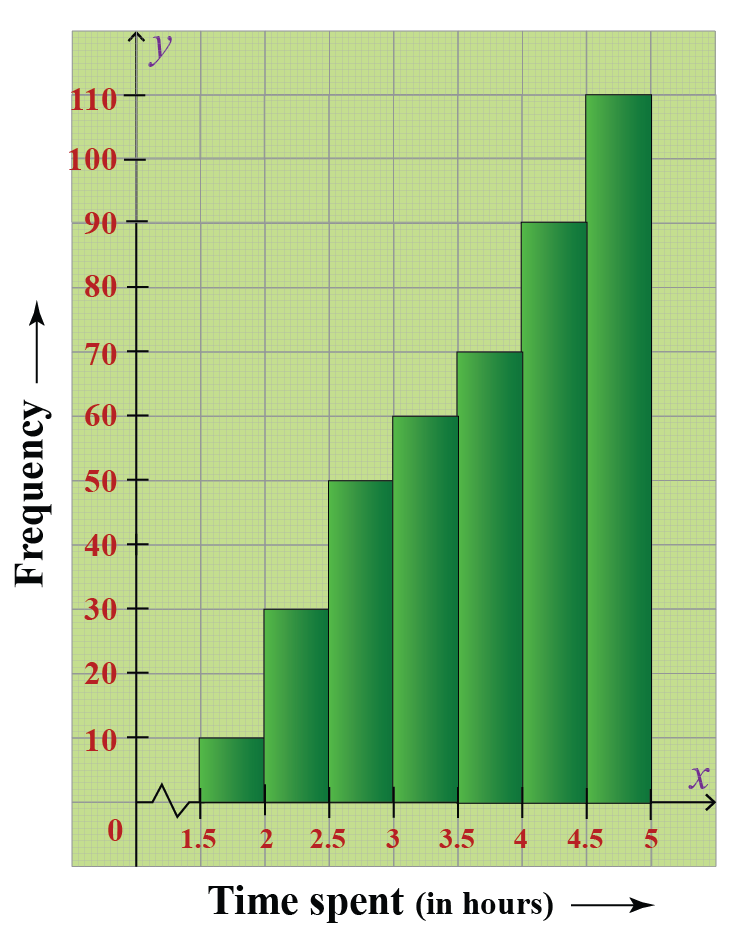Here the bars of the histogram is skewed to the left. Hence, it is a skewed left histogram.

### Uniform Histogram

A uniform histogram is a histogram where all the bars are more or less of the same height. It looks as follows: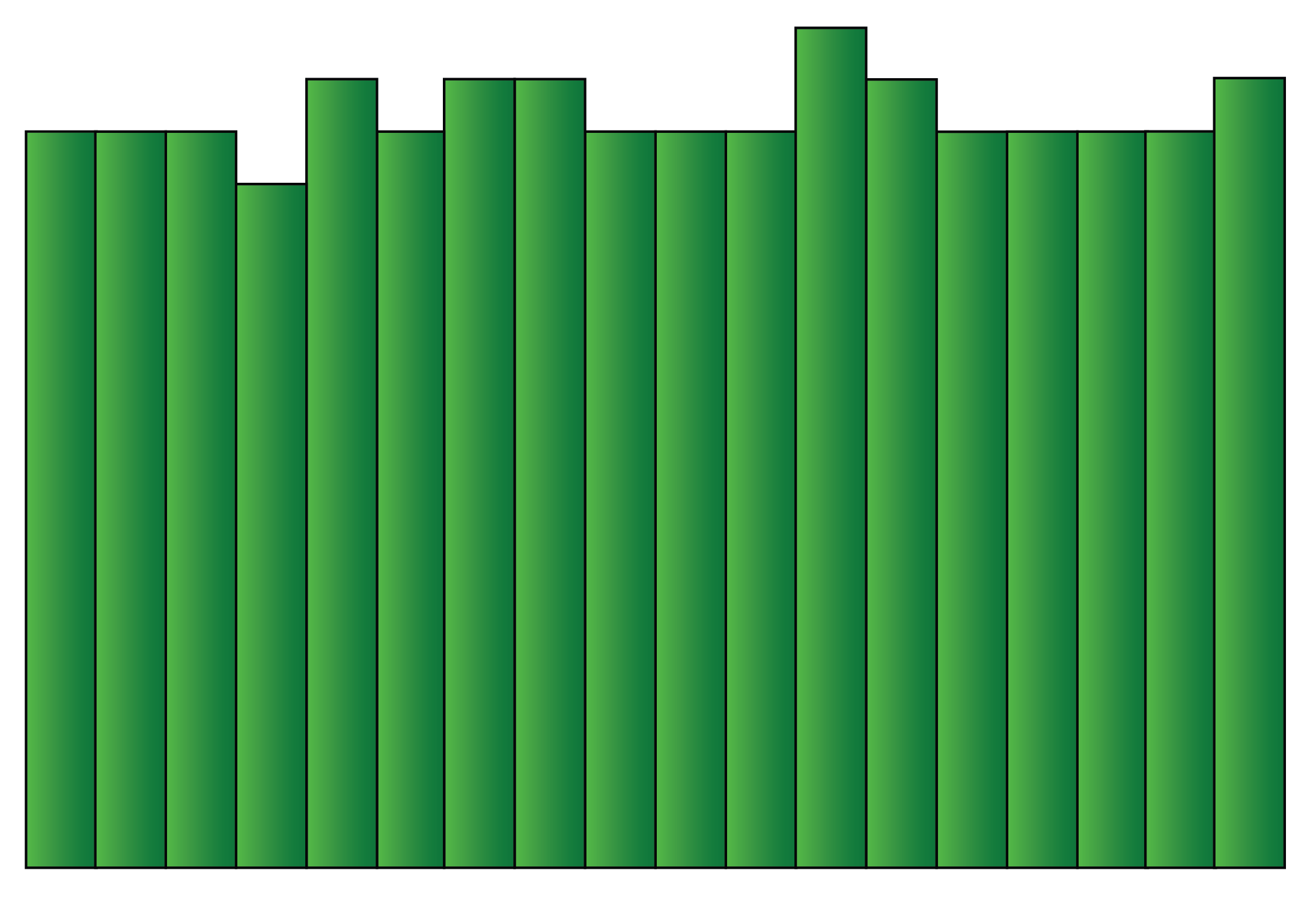Example:

Ma’am Lucy, the Principal of Little Lilly Playschool, wanted to record the heights of her students. The following histogram shows the number of students and their varying heights.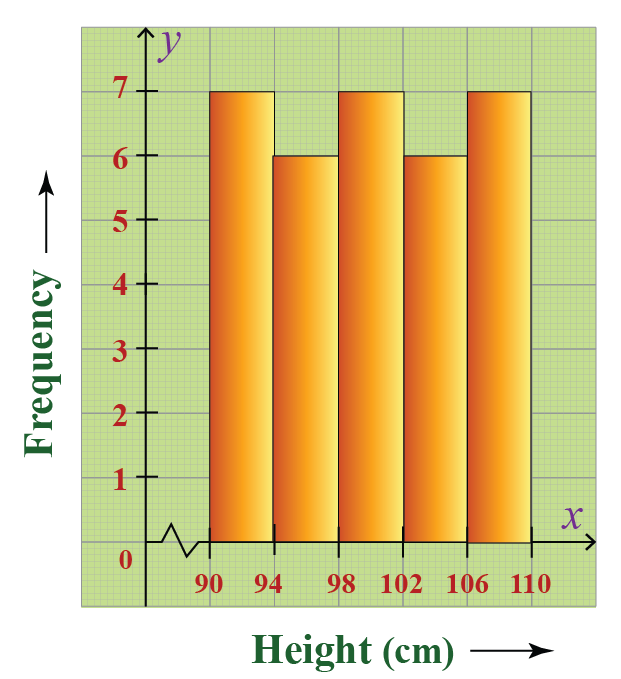The height of the students ranges between 90 cms to 110 cms.

In this histogram, the lengths of all the bars are more or less the same. Hence, it is a uniform histogram.

### Random Histogram

A random histogram is a histogram where the heights of bars have no pattern. It looks as follows: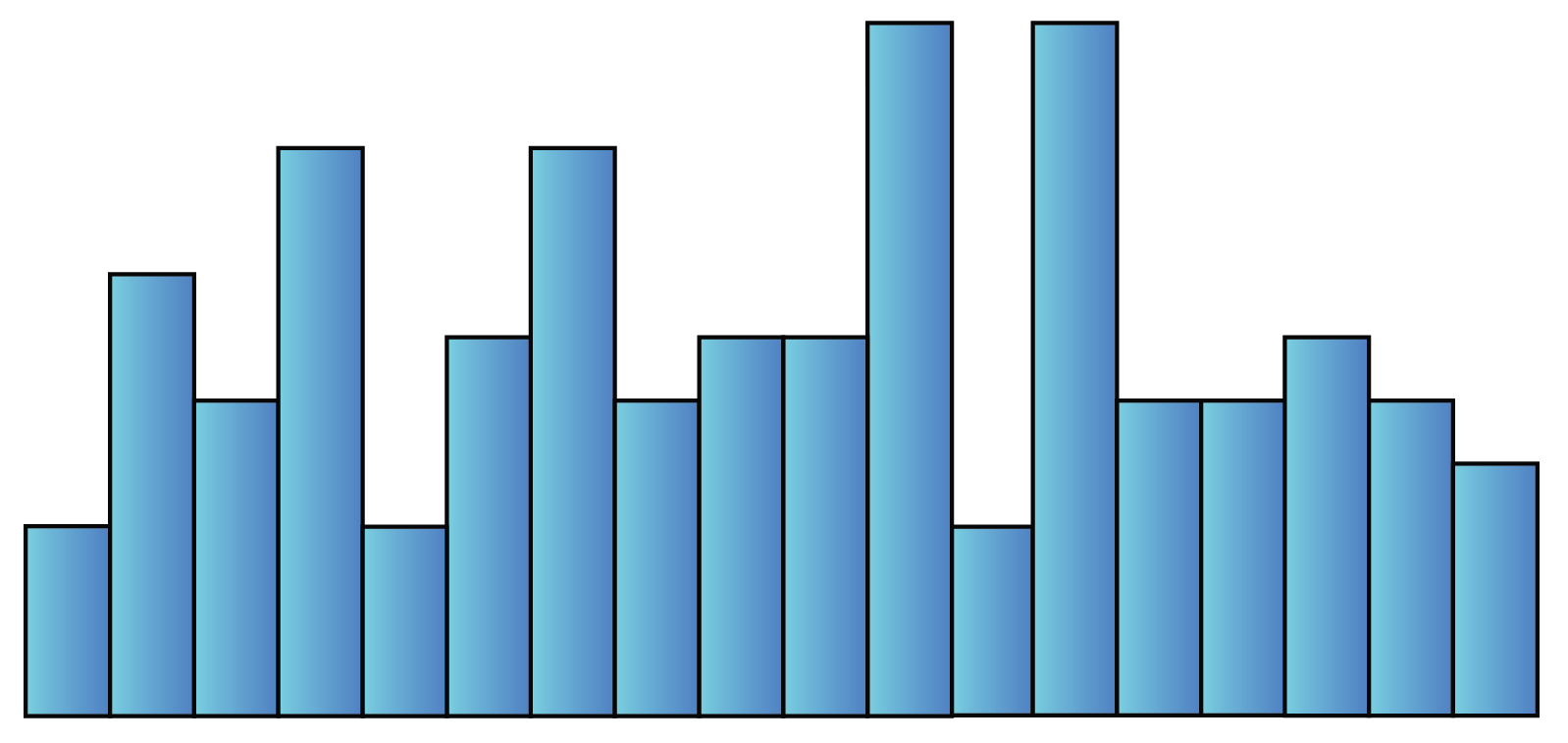Example:

The following histogram shows the different age groups of the people residing in J P Nagar, Bangalore.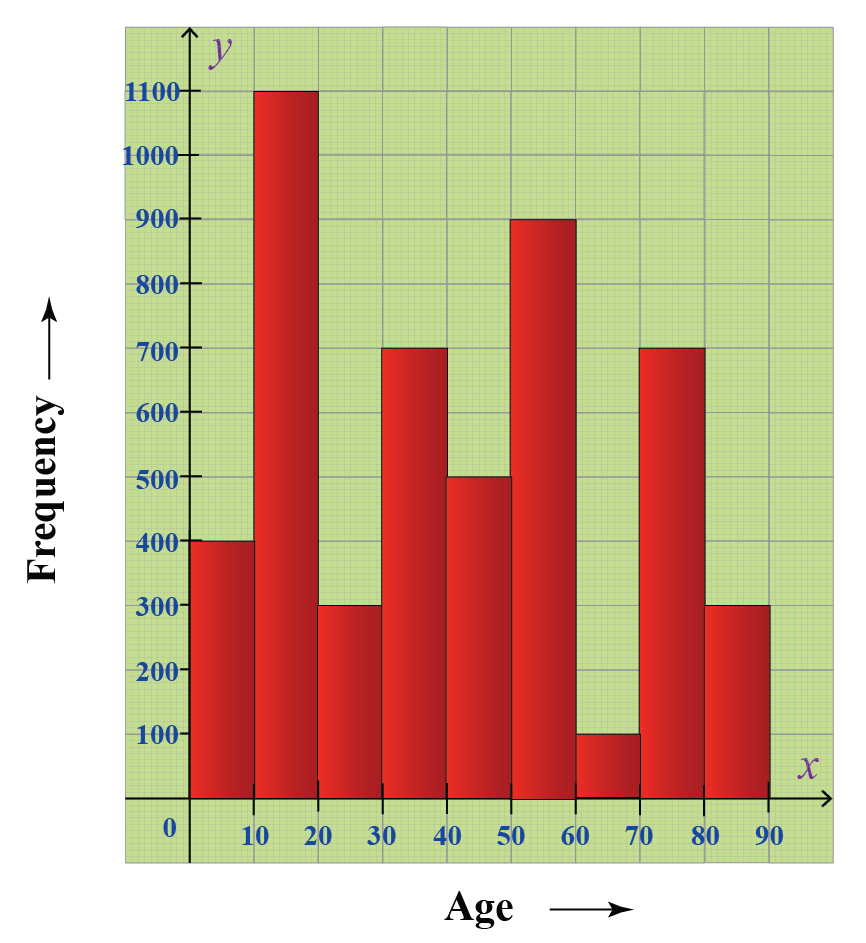The residents belong to varying age groups.

In this histogram, all bars are of different heights and there is no pattern. Hence, it is a random histogram.
Help your child score higher with Cuemath’s proprietary FREE Diagnostic Test. Get access to detailed reports, customised learning plans and a FREE counselling session. Attempt the test now.

## Difference Between a Bar Chart and a Histogram

• A bar graph is the graphical representation of categorical data using rectangular bars where the length of each bar is proportional to the value they represent.
• A histogram is the graphical representation of data where data is grouped into continuous number ranges and each range corresponds to a vertical bar.

The fundamental difference between histograms and bar graphs from a visual aspect is that bars in a bar graph are not adjacent to each other.

The main differences between a bar chart and a histogram are as follows:

Bar Graph Histogram
Equal space between every two consecutive bars. No space between two consecutive bars. They should be attached to each other.
X-axis can represent anything. X-axis should represent only continuous data that is in terms of numbers.

But in both graphs, Y-axis represents numbers only.

We can understand these differences from the following figure: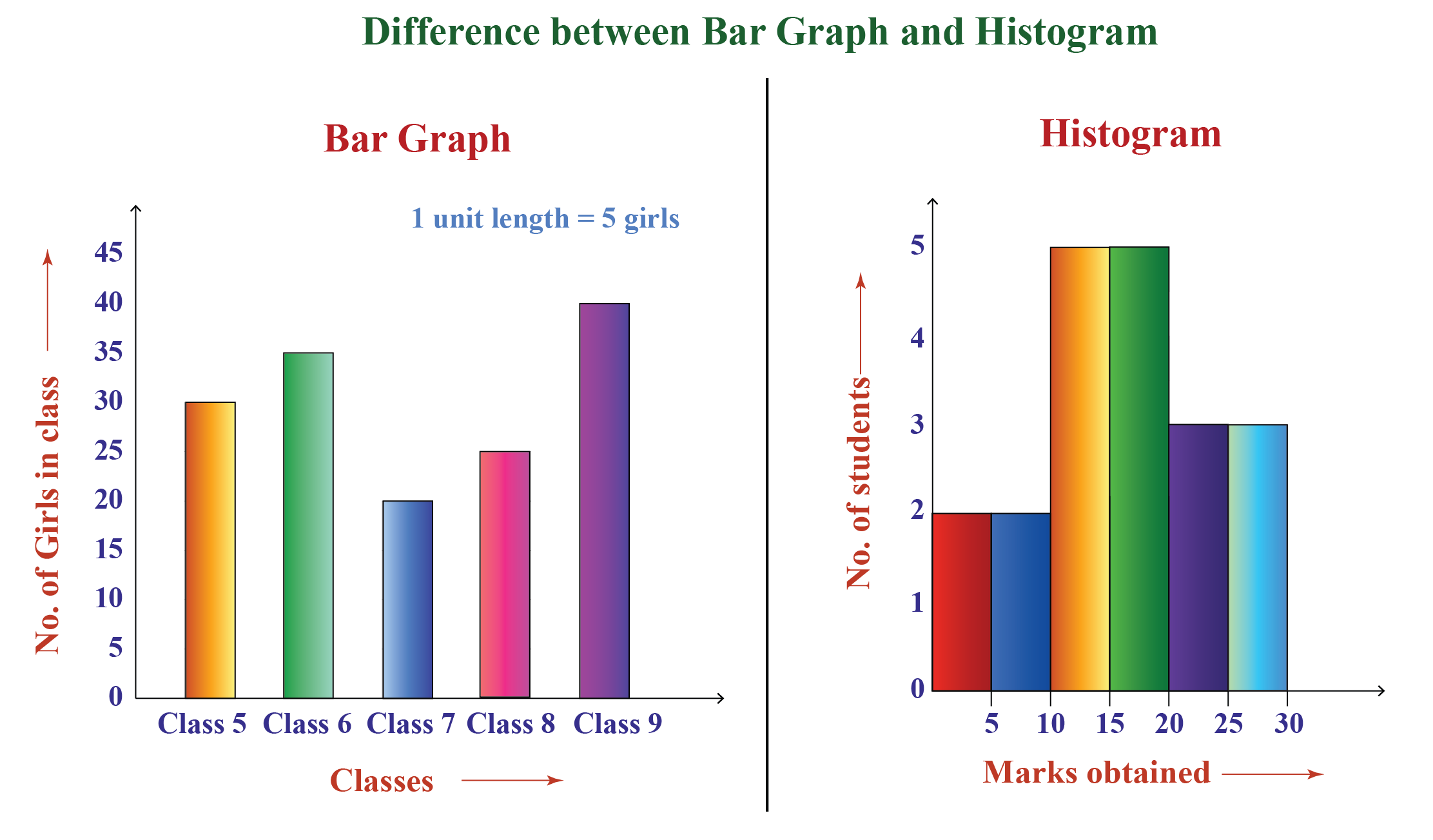## How to Calculate a Histogram?

The process of making a histogram using the given data is described using the following example:

Example:

Construct a histogram for the following frequency distribution table that describes the frequencies of weights of $$25$$ students in a class.

Weights (in Kg) Frequency (Number of students)
30 - 35 4
35 - 40 10
40 - 45 8
45 - 50 4

Steps to draw a histogram:

Step 1:

We choose a suitable scale to represent weights on the horizontal axis.
Here, we can choose the scale to be $$1 \text{ cm} = 5 \text{ kg}$$.
Since the weights in the table start from $$30$$, not from $$0$$, we give a break/kink on the X-axis.

Step 2:

We choose a suitable scale to represent the frequencies on the vertical axis.
Here we have frequencies varying from $$4$$ to $$10$$. Thus, we choose the scale to be $$1 \text{ cm} = 2$$.

Step 3:

We then draw the bars corresponding to each of the given weights using their frequencies.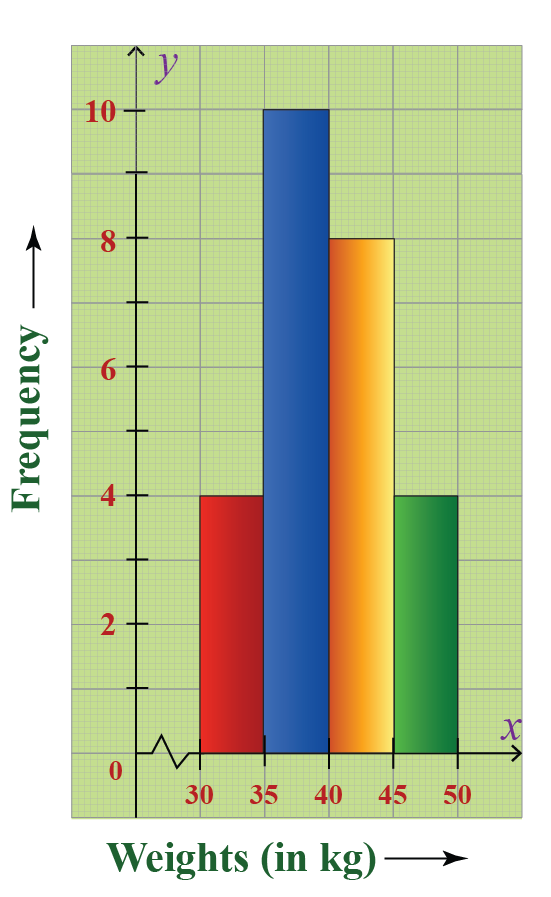Tips and Tricks
1. To choose the scale on the vertical axis while drawing histogram, check for the highest number that divides all the frequencies.
If there is no such number exists, then check for the highest number that divides most of the frequencies.

## Solved Examples

 Example 1

The blood groups of $$20$$ students of a class are recorded as follows:

A, B, O, O, B, A, O, O, O, AB, A, O, O, A, A, B, B, AB, O, B

Draw a frequency table to represent the given data and identify the rarest blood group among these $$20$$ students.

Solution:

In the given data, the number of students with blood group :

• $$A$$    = $$5$$
• $$B$$    = $$5$$
• $$O$$    = $$8$$
• $$AB$$ = $$2$$

The frequency distribution table of the data is:

Blood group Frequency
$$A$$  $$5$$
$$B$$  $$5$$
$$O$$  $$8$$
$$AB$$  $$2$$

From this table, the blood group with least frequency is $$AB$$.

 $$∴\text{The rarest blood group = AB}$$
 Example 2

Consider the following histogram that represents the weights of $$33$$ newborn babies in a hospital.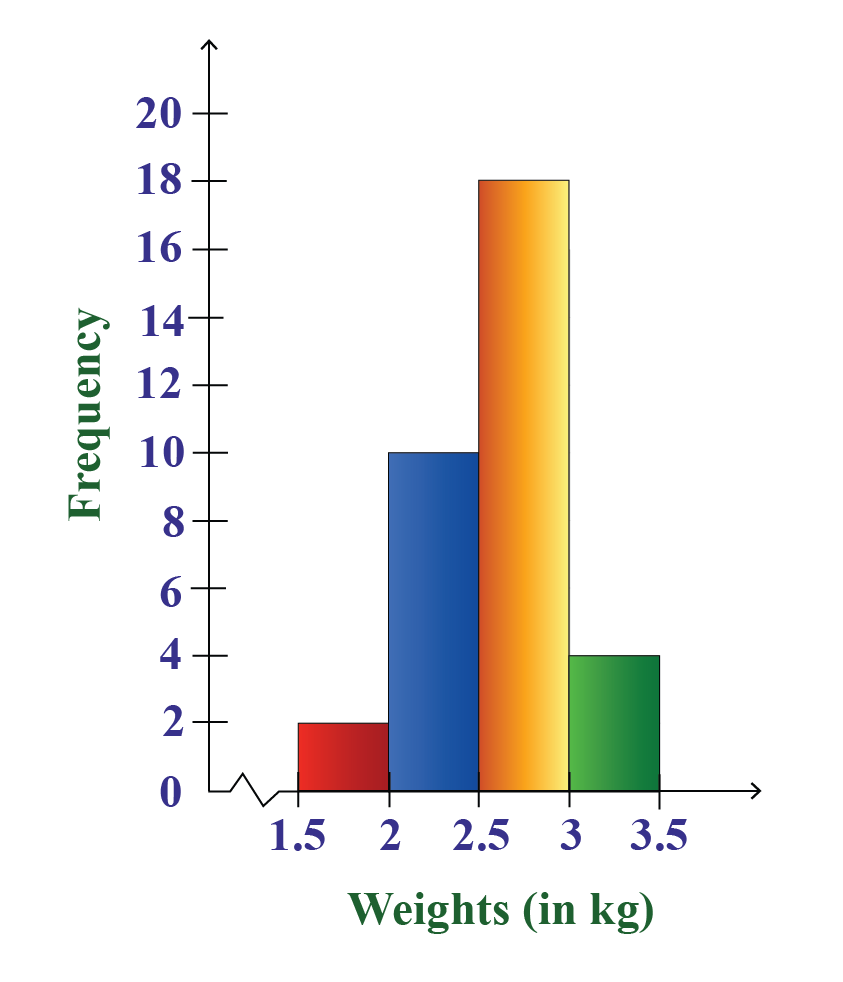If the children weighing between $$2$$ kg to $$3$$ kg are considered healthy, then find the percentage of the children of this hospital that are healthy.

Solution:

We have to first find the number of children weighing between $$2$$ kg to $$3$$ kg.

From the given histogram, the number of children weighing between:

• $$2 \text { kg } 2.5 \text { kg }$$ = $$10$$
• $$2.5 \text { kg } 3 \text { kg }$$ = $$18$$

Therefore, the number of children weighing between:

$2 \text{ kg} \text { to } 3 \text{ kg} = (10+18=28)$

The total number of children in the hospital = $$33$$

Hence, the required percentage is:
$\frac{28}{33} \times 100 \approx 85\%$

 $$∴\text{Required percentage} = 85\%$$
 Example 3

A random survey is done on the number of children belonging to different age groups who play in government parks and the information is tabulated as follows:

Age (in years) Frequency
$$1 - 2$$ $$8$$
$$2 - 4$$ $$10$$
$$4 - 7$$  $$18$$
$$7 - 9$$ $$10$$
$$9 - 11$$ $$12$$
$$11 - 15$$ $$6$$

(i) Draw a histogram representing the data.

(ii) Identify the number of children belonging to the age groups $$2, 3, 4,5,6$$ and $$7$$ who play in government parks.

Solution:

(i) We take the age (in years) on the horizontal axis of the graph and by observing the first column of the table, we choose the scale to be:

$1 \text{ cm} = 1 \text{ year}$

We take frequency on the vertical axis of the graph and by observing the second column of the table, we choose the scale to be:

$1 \text{ cm} = 2$

Now, we will draw the corresponding histogram.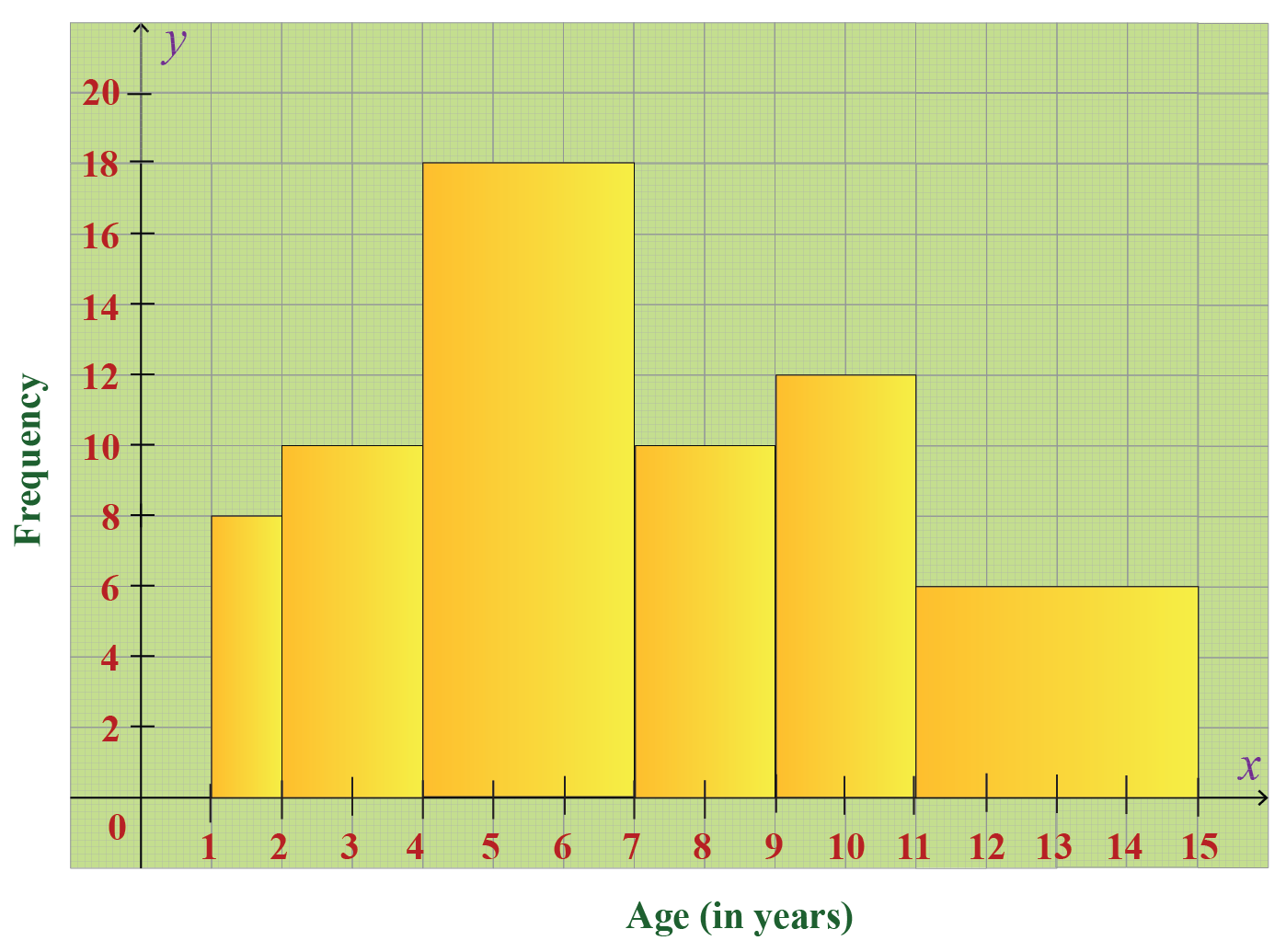(ii) From the table/graph, the number of children belonging to the age groups:

• $$2 \text { to } 4 \text { years }$$ = $$10$$
• $$4 \text { to } 7 \text { years }$$ = $$18$$

So the number of children belonging to the age groups $$2, 3, 4,5,6$$ and $$7$$ who play in government parks is

$10+18= 28$

 $$∴\text{Required number of children } = 28$$

More Important Topics
Numbers
Algebra
Geometry
Measurement
Money
Data
Trigonometry
Calculus